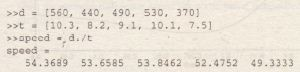Transportat on Route Analysis

The following table gives data for the distance traveled along five truck routes and the corresponding time required to traverse each route. Use the data to compute the average speed required to drive each route. Find the route that has the highest average speed.Solution

For example, the average speed on the first route is 560/ 10.3 = 54.4 mil hr. First we define the row vectors d and t from the distance and time data. Then, to find the average speed on each route using MATLAB, we use array division. The session isThe results are in’ miles per hour. Note that MATLAB displays more significant figures than is justified by the three-significant-figure accuracy of the given data. so we should I
round the results to three significant ~ . ~ figures before using them. Thus we should report the . average speeds to be 54.4, 53.7, 53.8, 52.5, and 49.0 mi/hr, respectively. To find t~e highest speed and the corresponding route, continue the session as follows:The first route hs,the highest speed. ‘ ;/ If we did not need the speeds for every route, we could have solved this problem by combining, to/.9/} irles as fiI 6ws: [highest_speed, route]’ = max (d. It). As
you oe ti1 me ‘;’ore familiar with MATLAB, you wilt-appreciate its power to solve problems with very few lines and keystrokes.